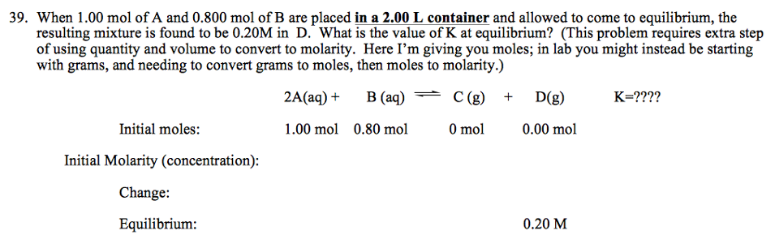# Problem: When 1.00 mol of A and 0.800 mol of B are placed in a 2.00 L container and allowed to come to equilibrium, the resulting mixture is found to be 0.20M in D. What is the value of K at equilibrium? (This problem requires extra step of using quantity and volume to convert to molarity. Here I'm giving you moles: in lab you might instead be starting with grams, and needing to convert grams to moles, then moles to molarity.)

###### FREE Expert Solution
88% (65 ratings)###### Problem Details

When 1.00 mol of A and 0.800 mol of B are placed in a 2.00 L container and allowed to come to equilibrium, the resulting mixture is found to be 0.20M in D. What is the value of K at equilibrium? (This problem requires extra step of using quantity and volume to convert to molarity. Here I'm giving you moles: in lab you might instead be starting with grams, and needing to convert grams to moles, then moles to molarity.)